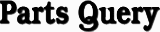Custom SearchANSWERS TO QUESTIONS Q1. THROUGH Q74. A1. Impedance decreases. A2. Degenerative feedback. A3. Transit time causes the grid voltage and plate current to be out of phase. A4. Transit time. A5. Velocity. A6. The electron will be accelerated. A7. By alternately speeding up or slowing down the electrons. A8. The buncher grids. A9. There is no effect. A10. The frequency period of the buncher grid signal. A11. Velocity modulation. A12. The accelerator grid and the buncher grids. A13. The catcher cavity. A14. Amplifier. A15. Intermediate cavities between the input and output cavities. A16. A large negative pulse is applied to the cathode. A17. The middle cavity. A18. The bandwidth decreases. A19. Stagger tuning. A20. The reflector or repeller. A21. Velocity. A22. Three-quarter cycle. A23. Mode 2. A24. Power is reduced. A25. The half-power points of the mode. A26. Voltage amplification. A27. Used to focus the electrons into a tight beam. A28. The directional couplers are not physically connected to the helix. A29. The traveling wave must have a forward velocity equal to or less than the speed of the electrons in the beam. A30. The helix. A31. Helix. A32. A magnetic field. A33. Anode or plate. A34. The resonant cavities. A35. The permanent magnet. A36. The critical value of field strength. A37. Circular. A38. The negative-resistance magnetron has a split plate. A39. The application of the proper magnetic field. A40. To reduce the effects of filament bombardment. A41. Rising-sun block. A42. Series. A43. Working electrons. A44. Greater power output. A45. Loops and slots. A46. Inductive. A47. A cookie-cutter tuner. A48. Baking in. A49. The tunneling action. A50. The tuned circuit or cavity frequency. A51. To increase the stability. A52. Prevent feedback to the tuned input circuit. A53. Stability problems. A54. Variable capacitor. A55. Reactance. A56. The low-noise characteristic. A57. By varying the amount of capacitance in the circuit. A58. Supplies the electrical energy required to vary the capacitance. A59. Exactly double the input frequency. A60. The pump signal of a nondegenerative parametric amplifier is higher than twice the input signal. A61. Idler- or lower-sideband frequency. A62. The sum of the input frequency and the pump frequency. A63. Larger microwave power outputs. A64. The electrons become immobile. A65. Threshold. A66. A field of much greater intensity. A67. The frequency. A68. Pnin. A69. The negative-resistance property. A70. To form a small region of p-type material. A71. Lower. A72. Lower forward resistance and low noise. A73. Variable resistance. A74. A switching device.Integrated Publishing, Inc. - A (SDVOSB) Service Disabled Veteran Owned Small Business